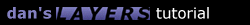2.2 Sine Wave

A sine wave is very similar to the hover and circle. The movement along the horizontal is a constant value, but it is related to the wavelength. This made it a little tricky but I got it to work.

```function sine() {
SinLayername = "layer1";   // name of the layer you want to move
SinAmplitude = 120;        // amplitude of wave in pixels (remember you go up AND down this amount)
SinWavelength = 300;       // wavelength in pixels
SinCycles = 1;             // the number of times you want to cycle through the wave (decimals allowed)
SinAngleinc = 5;           // angle incrementation
SinAnglestart = 0;         // angle you want to start the wave at
SinDirection = 1;          // use "1" to move right, "-1" to move left
SinSpeed = 20;             // speed of repetition in milliseconds
SinAngle = SinAnglestart;
SinLeft = document.layers[SinLayername].left;
SinTop = document.layers[SinLayername].top;
slidesine();
}

function slidesine() {
if (Math.abs(SinAngle) < SinCycles*360) {
var x = SinLeft + SinDirection*(SinAngle / (360/SinWavelength)) - SinDirection*(SinAnglestart / (360/SinWavelength));
var y = SinTop - (SinAmplitude/2 * Math.sin(SinAngle*Math.PI/180)) + (SinAmplitude/2 * Math.sin(SinAnglestart*Math.PI/180));
document.layers[SinLayername].moveTo(x,y);
SinAngle += SinAngleinc;
setTimeout("slidesine()",SinSpeed);
}
}
```1.1 Introduction 1.2 Overlapping 1.3 Nesting 1.4 Using JavaScript 2.1 Sliding Layers 2.2 Pre-Built Functions 2.3 Clipping Layers 2.4 Looping Animations 2.5 Changing Images 3.1 Mouse-Click Animation 3.2 Capturing Keystrokes 3.3 Drag and Drop 4.1 Making Demos 4.4 Problems 4.5 Screen Sizes Copyright © 1997 Dan Steinman. All rights reserved.